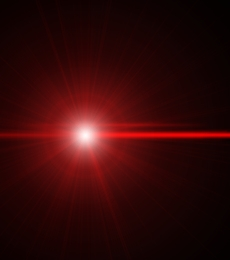# Two-Photon AbsorptionThe page on linear absorption & scattering describes the effects when an individual photon has the right energy to excite atmospheric molecules, and single photon ionization is about when individual photons have enough energy to knock an electron off an atom. You can also get multi-photon absorption and ionization, where the simulatneous absorption of many photons provides enough energy to create the excitation. Because all the photons must participate at once, it requires much higher light intensities. In general, for absorption of N photons at once, the rate of absorption will be proportional to intensity raised to the Nth power.
 dI = -αN IN dx

As the intensity of the death ray rises, the first such non-linear effect to occur is two photon absorption. This gives the differential equation
 dI = -α2 I2 dx
The power P of a beam is equal to the beam area A times its intensity I, the beam energy E is equal to its power times its duration δt. This gives us
 dP = -α2 P2 dx A
For constant beam area and initial power P0, this has the solution
 P(x) = P0 1 + α2 x P0 ⁄ A

For a focused beam with a focal point at xf, the area changes with x as
 A(x) = A0 ( xf-x )² xf
until the beam get close to its diffraction-limited area. As a rough approximation, the beam can be considered to converge according to the above formula until it reaches its diffraction limited area Ad, and then propagate with its area equal to its diffraction limited area for a distance of xd = Ad/λ, where λ is the beam's wavelength.

For focused beams, while the beam is still converging the differential equation for power loss gives
 P(x) = P0 1 + (α2 xf P0 ⁄ A0)[x ⁄ (xf - x)]

The rapidly increasing intensity as you approach the focus means that if
 α2 xd P0 > 1 Ad
the beam will rapidly lose power to two-photon absorption and very little of the original power can be expected to be incident on the target.